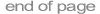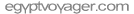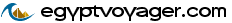<< Back           hieroglyph : lesson 7

 Lesson VII : Numbers and dates

1) Whole numbers and fractions.

The Ancient Egyptian numeric system consisted of a sign for units, and special signs for the various powers of ten.

 UnitsTens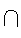Hundreds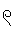Thousands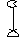Tenthousands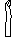Hundredthousands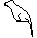Millions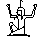Whole numbers were written by using as many of these signs as needed to make up the total number, starting with the highest. Thus the number 5 was written by repeating the unit-sign 5 times: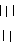; 50 by repeating the sign for "tens" 5 times: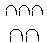; and 55 by repeating the sign for "tens" 5 times, followed by 5 times the unit-sign: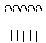. The latter could be interpreted as (5 times 10) + (5 times 1) = 55.

Care must be taken not to confuse the number 1 with the determinative stroke mentioned in Lesson IV.

The sign for million, which also means "many" and "infinity", early fell into disuse. Higher numbers and values were sometimes written in a different way: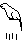means 4 times 100,000 = 400,000.

The numeric system did not include a decimal point. Decimal numbers were written as fractions. With the exception of 1/2, 2/3 and 3/4, fractions were always written using the sign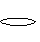, combined with a whole number, to convey the meaning 1/x. E.g.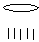means 1/5. This example can be transcribed both as r-5 and as 1/5.

Fractions with numerators bigger than 1 were written as a sum of fractions with numerators equal to 1. Thus 2/5 was written as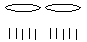. Complex fractions were always broken down to the simplest sum of 1/x type fractions. 3/8 was written as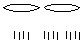, 1/4 + 1/8 and not by repeating the group for 1/8 three times.

The already mentioned exceptions to this rule are 1/2, which is written as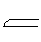, 2/3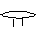and 3/4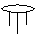.

2) The use of numbers to indicate amounts.

Amounts were written after the word of which they render the amount. The word to which the number is added, is normally written in singular. Some examples follow: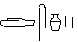ds 2, "two jugs"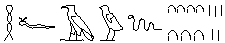HfAw 75, "75 snakes"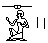s 2, "two men"...

3) Dates

The Ancient Egyptians used three different kinds of calendars: an agricultural, a lunar and an astronomical. The latter two were mainly used for liturgical purposes and were mostly limited to temples. Thus the lunar calendar was used to make specific rituals for lunar gods, such as Khonsu, coincide with specific lunar phases.

The agricultural calendar, on the other hand, was used to date all kinds of events, documents, … It divided the year into 3 seasons of 4 months: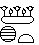Ax.t, the season of inundation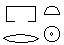pr.t, the season of sowing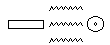Smw, the season of harvesting (summer).

Although months had names they were only rarely used in dates. Most often, months numbered from the start of each season on; e.g.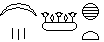ibd 3 (n) Ax.t, "the third month of Akhet" or "the third month of inundation". The word for month is transcribed ibd or Abd and was written using a sign that represents a part of the moon.

Each month was divided into 30 days. Days were counted from the beginning of each month on. E.g.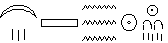ibd 3 (n) Smw ssw 25, "the 3rd month of Shemu, the 25th day". The word for "day" in dates can be either ssw or hrw. When it is only written using the sign that represents the solar disk, one can chose between either two of them.

The Egyptian year thus counted 12 months of 30 days, or 360 days in total, to which 5 so-called "epagomenal" days were added to make the year correspond more or less to the solar cycle.

In theory, the first day of the first month of Ax.t was supposed to coincide with the start of the annual inundation of the Nile. There were no leap years, so the agricultural calendar lacked one day every four years. For this reason, the 1st day of the 1st month of Ax.t could fall on any day of our calendar.

From the Middle Kingdom on, years were numbered starting the accession to the throne of a new king. A regnal year was written as, HA.t-sp, followed by the number of that year; e.g.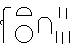, HA.t-sp 15. The regnal year can be followed by a more precise date, following the agricultural calendar, and the name of a king. The name of the king could simply be his prenomen or his nomen, but it could also be his full titulary.

E.g.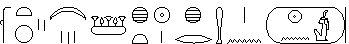HA.t-sp 2, ibd 3 (n) Ax.t, ssw 1 xr Hm n (n-mAa.t-ra), Year 2, the 3rd month (of) Akhet, the first day under the Majesty of (Ni-Maat-Re).